# Engineering Mechanics - Force Vectors

### Exercise :: Force Vectors - General Questions

• Force Vectors - General Questions
11.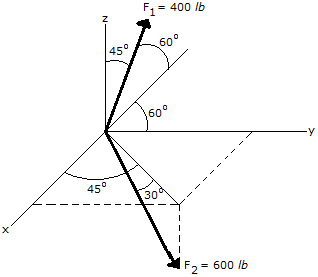Express the force F1 in Cartesian vector form.

 A. F1 = (200 i - 200 j + 283 k) lb B. F1 = (200 i + 200 j + 283 k) lb C. F1 = (-200 i + 200 j + 565 k) lb D. F1 = (-200 i + 200 j + 283 k) lb

Explanation:

No answer description available for this question. Let us discuss.

12.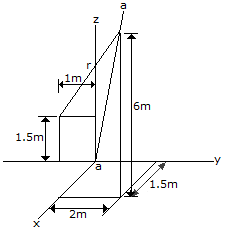Determine the projection of the position vector r along the aa axis.

 A. raa = 35.3 m B. raa = 6.28 m C. raa = 5.42 m D. raa = 5.61 m

Explanation:

No answer description available for this question. Let us discuss.

13.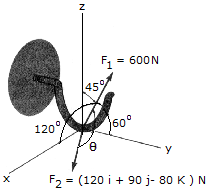What is the projection of the force F2 along the positive axis?

 A. F2y = 170.0 N B. F2y = —80.0 N C. F2y = 90.0 N D. F2y = 120.0 N

Explanation:

No answer description available for this question. Let us discuss.

14.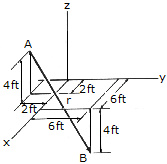Determine the magnitude and direction of the position vector r which points from point A to point B.

 A. r = 12 ft,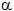= 70.5°,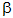= 48.2°,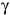= 131.8° B. r = 12 ft,= 109.5°,= 131.8°,= 48.2° C. r = 12 ft,= 70.5°,= 48.2°,= 48.2° D. r = 12 ft,= 109.5°,= 131.8°,= 131.8°

Explanation:

No answer description available for this question. Let us discuss.

15.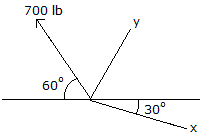Determine the magnitude of the x and y components of the 700-lb force.

 A. Fx = -350 lb, Fy = 606 lb B. Fx = -404 lb, Fy = 571 lb C. Fx = -606 lb, Fy = 350 lb D. Fx = -571 lb, Fy = 404 lb